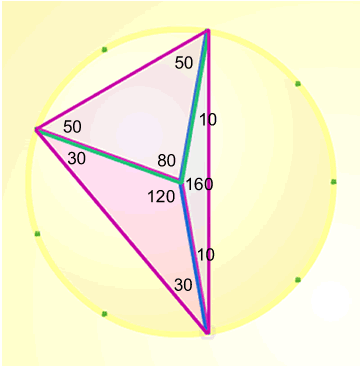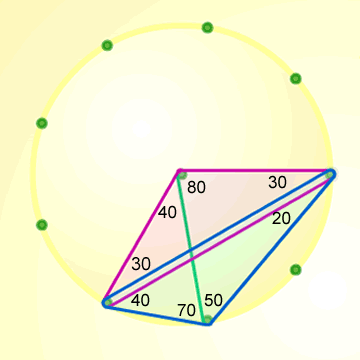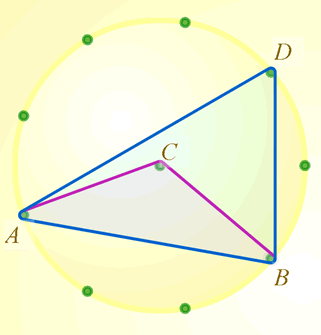#### You may also like### On Time

On a clock the three hands - the second, minute and hour hands - are on the same axis. How often in a 24 hour day will the second hand be parallel to either of the two other hands?### Estimating Angles

How good are you at estimating angles?### LOGO Challenge 1 - Star Square

Can you use LOGO to create this star pattern made from squares. Only basic LOGO knowledge needed.

# Subtended Angles

### Why do this problem?

This problem leads towards the theorem about the relationship between the angle subtended by ("hanging from") an arc at the centre of a circle and the angle subtended by the same arc at the circumference. It's worth noting that once students know this relationship, they will be able to deduce that angles subtended by an arc at the circumference are all equal.

The nine-peg circle allows students to concentrate on the geometrical structure without having to worry about the arithmetic. A special case of this problem is Right angles.

Teachers may find the article Angle Measurement: an Opportunity for Equity of interest.

### Possible approach

All students will need a sheet of nine-peg circles to jot down ideas during discussion.

Demonstrate how the geoboard works - clicking on a coloured rubber band, dragging it onto a peg and then "stretching" it out onto two more pegs to make a triangle.

Draw a triangle that encloses the centre, split it into three isosceles triangles and find the angles.
An example may look like this:"The angles around the centre add up to 360 degrees and the angles at the vertices add up to 180 degrees. What else do you notice about angles at the centre and angles at the vertices?"

Ask students to take a look at their working for Triangles in Circles to identify general rules that work in all cases. Encourage students to consider all the different possible angles at the circumference subtending a specific arc.

When the angle at the centre is a reflex angle, students may need some help to see that the angle at the circumference is still half the angle at the centre. For example:A Virtual Geoboard allows you to create triangles in circles with a variable number of pegs. Ask students to check that the relationship between angles at the centre and angles at the circumference holds in 10 peg, 12 peg, 15 peg ... circles.

### Key questions

• What is the relationship between the angle at the centre and the angle at the circumference?

• What do we know already that might be useful here?

• What are the implications of our findings? (How can other circle theorems be deduced from this one?)

### Possible extension

• Would the same thing happen if you started with an arc between any two points on the circumference of any circle?

• Can you prove it?

### Possible support

Students can work with several ready-made triangles like the one below and asked to compare angles ACB and ADB. The sheet of nine-peg circles could be used to draw these.